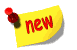Admissions for B.Sc. First year, B.C.A. First year and M.Sc. First year Maths are in full swing Date: 8/06/2023 More InfoAll the stake holders of Bhalerao Science College are advised to go through the website for any errors, additions and deletion of data and send confirmation message on college email. Date: 11-May-2022 More Info

# MRP Dr. Salve

FINAL REPORT OF THE WORK DONE ON THE PROJECT

1. NAME AND ADDRESS OF THE PRINCIPAL INVESTIGATOR …

Miss. Pravina Madhukar Salve, Dept. Mathemaics, Bhalerao Science College, Saoner, Dist. Nagpur

2. NAME AND ADDRESS OF THE INSTITUTION …

Bhalerao Science College, Saoner, Dist. Nagpur

3. UGC APPROVAL NO. AND DATE …23-1777/10(WRO) Dated: 14/ 10 /2010

4. DATE OF IMPLEMENTATION ……15/02/2011

5. TENURE OF THE PROJECT ……Two years

6. TOTAL GRANT ALLOCATED …  Rs.75,000/- (Rs. Seventy Five Thousand)

7. TOTAL GRANT RECEIVED ……  Rs.55,000/- (Rs. Fifty Five Thousand)

8. FINAL EXPENDITURE …………   Rs.37,854/- (Rs. Seventy Five Thousand)

9. TITLE OF THE PROJECT ……

"Study of Heat Conduction in Thermoelasticity, A Boundary Value Problem"

10. OBJECTIVES OF THE PROJECT …

- Formulation of Heat Conduction problem on various solids and study of its thermoelasticity.

- Further study of the existing methods.

- Construction of new methods.

11. WHETHER OBJECTIVES WERE ACHIEVED (GIVE DETAILS)

……The study was carried out for the cylindrical solids. The problem of thermoelasticity was studied over two different solids, one example is of Hollow cylinder and the other is of Concentric cylinder. The methods were formulated for the thermoelasticity problem by considering the boundary value conditions. The approach is made towards obtaining the theoretical solution of the problem and the mathematical software MATHCAD was used to obtain the solutions of the different equations so that the graphical presentation can be sort out. Thus the objectives were achieved.

12. ACHIEVEMENTS FROM THE PROJECT …………Two research papers were published out of which one is in the International journal of repute..

13. SUMMARY OF THE FINDINGS ……

Summary of the findings.. According to the proposal submitted for minor research paper the study of heat conduction in thermoelasticity under boundary value conditions for different solids were carried out. Here the study was carried out on the two different solids. One is the Concentric cylinder and the other is the Hollow cylinder. In the first example:-“Transient heat conduction in a concentric cylinder and its thermal stresses”, the solution of the problem of transient heat conduction in a concentric cylinder, subjected to heating of the concentric cylinder with its lower surface kept as arbitrary and upper surface kept as zero, is obtained by employing the integral transform technique in the form of infinite series for which two alternative forms, suitable for detailed numerical computation under different boundary conditions are given by introducing the so called pseudo-steady temperature distribution function.

Applying finite Hankel transform, finite Fourier transform, finite Laplace transform and its inverse to find out the General and alternate solution of a concentric cylinder.

The calculations have been carried out using a computer software MathCAD and the graphs have been plotted, there are some different and some similar patterns observed in the graphs.

The numerical results are presented for the time variation of the inferred heating temperature distribution, the displacement and stresses. The variation of temperature distribution T/a, displacement y/b, and stresses srr/g and sqq/g  for different cases are represented graphically.

In the secondexample:-“steady-state thermoelastic problem of a finite length hollow cylinder” for determination of steady state thermoelasticity in finite length of hollow cylinder with the temperature maintained at zero on the upper surface of the plate and a third kind boundary condition maintained at arbitrary constant f(r) on the lower surface of the plate. Here, in this example, an attempt is being made to find the solution of the steady state thermoelasticity in finite length of hollow cylinder with its outer edges subjected to the third kind boundary condition as zero. In order to obtain the solution of the governing equation, which is a partial differential equation, the following procedures of analysis have been adopted.

1. Normalizing of the governing partial differential equation subject to appropriate initial, boundary and interior condition.

2. Taking finite Hankel transform of the resulting equation with respect to variable r.

3. Achieving the inverse finite Hankel transform. The expression of temperature distribution, thermoelastic displacement and stress functions are obtained in terms of Bessel’s function.

Equations are calculated using MathCad, and graphs have been plotted, for temperature distribution T, thermoelastic displacement f, radial displacement U, axial displacement W, and stress functions sr, sz, sq, trz, of the hollow cylinder versus radius for different values of z. In the same way, graphs have been plotted for different values of radius r versus z. The above results shown in these plots are the Quasi-static thermoelastic state deformation of the finite length of the hollow cylinder.

PRINCIPAL INVESTIGATOR …